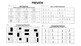# FractionsSubject
Resource Type
Format
PDF (842 KB|14 pages)
Standards
\$8.00
• Product Description
• Standards

Skills - 14 Resources that cover the following topics. Can be used for exit tickets, centers, daily work.

1. Equal Parts
2. Drawing Fractions
3. Identifying Fractions
4. Fractions on a Number Line
5. Unit Fractions
6. Sum of Unit Fractions
7. Equivalent Fractions
8. Comparing Fractions
9. Equal Shares
to see state-specific standards (only available in the US).
Compare two fractions with the same numerator or the same denominator by reasoning about their size. Recognize that comparisons are valid only when the two fractions refer to the same whole. Record the results of comparisons with the symbols >, =, or <, and justify the conclusions, e.g., by using a visual fraction model.
Express whole numbers as fractions, and recognize fractions that are equivalent to whole numbers. Examples: Express 3 in the form 3 = 3/1; recognize that 6/1 = 6; locate 4/4 and 1 at the same point of a number line diagram.
Recognize and generate simple equivalent fractions, (e.g., 1/2 = 2/4, 4/6 = 2/3). Explain why the fractions are equivalent, e.g., by using a visual fraction model.
Understand two fractions as equivalent (equal) if they are the same size, or the same point on a number line.
Explain equivalence of fractions in special cases, and compare fractions by reasoning about their size.
Total Pages
14 pages
N/A
Teaching Duration
N/A
Report this Resource to TpT
Reported resources will be reviewed by our team. Report this resource to let us know if this resource violates TpT’s content guidelines.
• Ratings & Reviews
• Q & A

Teachers Pay Teachers is an online marketplace where teachers buy and sell original educational materials.